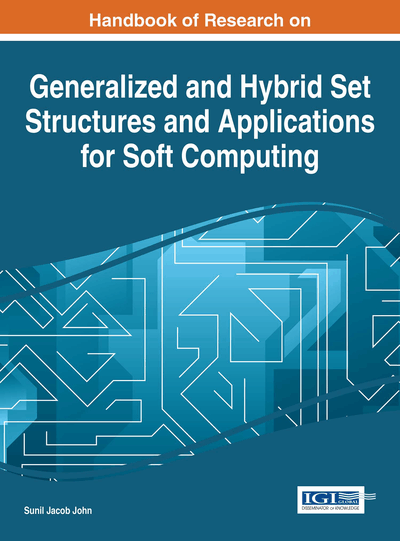# Soft Sets and Its Applications

B. K. Tripathy (VIT University, India) and K. R. Arun (VIT University, India)
DOI: 10.4018/978-1-4666-9798-0.ch005

## Abstract

Uncertainty is an inherent characteristic of modern day databases. In order to handle such databases with uncertainty, several new models have been introduced in the literature. Some new models like fuzzy sets introduced by Zadeh (1965), rough sets invented by Z. Pawlak (1982) and intuitionistic fuzzy sets extended by K.T. Atanassov (1986). All these models have their own pros and cons. However, one of the major problems with these models is the lack of sufficient number of parameters to deal with uncertainty. In order to add adequate number of parameters, soft set theory was introduced by Molodtsov in 1999. Since then the theoretical developments on soft set theory has attracted the attention of researchers. However, the practical applications of any theory are of enough importance to make use of it. In this chapter, the basic definitions of soft set, operations and properties are discussed. Also, the aim in this chapter is to discuss on the different applications of soft sets; like decision making, parameter reduction, data clustering and data dealing with incompleteness.
Chapter Preview
Top

## 3. Definitions And Concepts

In this section, we shall introduce several definitions including that of soft set and operations on them are to be presented.

• Definition (Soft set): Let U is a classical set of elements, the pair (U, E) is called the universe and E be a set of parameters often regarded as a soft universe. Members of the universe and the parameter set are generally denoted by x and e respectively. A soft set over the soft universe (U, E) is denoted by (F, A), where F:AP(U), where A is a subset of E and P (U) is the power set of U which comprises of all the subsets of U.

## Key Terms in this Chapter

Parameter Reduction: Reducing the number of parameters using any techniques and core parameter is identified for easy manipulation.

Data Clustering: The process of putting a given data set into groups of similar elements is called data clustering.

Soft Set: soft set was introduced by Molodtsov(1999) . Let U is a classical set of elements, the pair (U, E) is called the universe and E be a set of parameters often regarded as a soft universe. Members of the universe and the parameter set are generally denoted by x and e respectively. A soft set over the soft universe (U, E) is denoted by (F, A), where F : A ? P ( U ), where A is a subset of E and P (U) is the power set of U which comprises of all the subsets of U.

Rough Set: Rough set theory was initiated by Pawlak (1982). Let U be a universe and R be an equivalence relation over U. This equivalence relation decomposes U into disjoint equivalence classes. We denote the equivalence class of an element x with respect to R by [ x ] R , which is defined as [ x ] R = { y | yRx }. Then for any , we associate two crisp sets and called the lower and upper approximations of X with respect to R respectively and are defined as, = { x ? U: X} and = { x ? U: [ x ] R n X }.

Fuzzy Set: Fuzzy set was introduced by Zadeh (1965). Fuzzy set is the extension of crisp set. In fuzzy, each and every element has the degrees of membership value which lies between [0, 1]. A fuzzy set is the pair ( S , µ) where S is a set and µ : S ? [0,1].

Intuitionistic Fuzzy Set: An (IFS) A over E, is characterised by two functions µ A and ? A called the membership and non-membership function of A respectively such that µ A :U ? [0, 1] and ? A :U ? [0, 1]. For any x ? U , we have 0 = µ A ( x ) + ? A ( x ) = 1. The hesitation function of A is denoted by p A and for any x ? U , is given by p A ( x ) = 1 – µ A ( x ) – ? A ( x ). It is the indeterministic part of x.

Incomplete Soft Set: Missing of some data in the soft set due to the incomplete database exist in literature.

## Complete Chapter List

Search this Book:
Reset